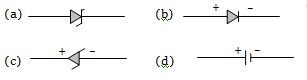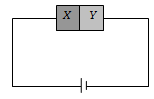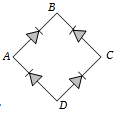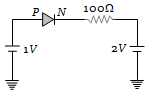The correct symbol for zener diode isConcept Questions :-

Applications of PN junction

Difficulty Level:

Which one of the following statements is not correct

(a) A diode does not obey Ohm's law

(b) A PN junction diode symbol shows an arrow identifying the direction of current (forward) flow

(c) An ideal diode is an open switch

(d) An ideal diode is an ideal one way conductor

Concept Questions :-

PN junction
High Yielding Test Series + Question Bank - NEET 2020

Difficulty Level:

A semiconductor X is made by doping a germanium crystal with arsenic (Z = 33). A second semiconductor Y is made by doping germanium with indium (Z = 49). The two are joined end to end and connected to a battery as shown. Which of the following statements is correct(a) X is P-type, Y is N-type and the junction is forward biased

(b) X is N-type, Y is P-type and the junction is forward biased

(c) X is P-type, Y is N-type and the junction is reverse biased

(d) X is N-type, Y is P-type and the junction is reverse biased

Concept Questions :-

Types of semiconductors
High Yielding Test Series + Question Bank - NEET 2020

Difficulty Level:

Which is the wrong statement in following sentences?

A device in which P and N-type semiconductors are used is more useful then a vacuum type because

(a) Power is not necessary to heat the filament

(b) It is more stable

(c) Very less heat is produced in it

(d) Its efficiency is high due to a high voltage across the junction

Concept Questions :-

Applications of PN junction

Difficulty Level:

In the diagram, the input is across the terminals A and C and the output is across the terminals B and D, then the output is(a) Zero
(b) Same as input
(c) Full wave rectifier
(d) Half wave rectifier

Concept Questions :-

Rectifier
High Yielding Test Series + Question Bank - NEET 2020

Difficulty Level:

The current through an ideal PN-junction shown in the following circuit diagram will be(a) Zero
(b) 1 mA
(c) 10 mA
(d) 30 mA

Concept Questions :-

PN junction
High Yielding Test Series + Question Bank - NEET 2020

Difficulty Level:

If a full wave rectifier circuit is operating from 50 Hz mains, the fundamental frequency in the ripple will be

(a) 50 Hz                           (b) 70.7 Hz
(c) 100 Hz                         (d) 25 Hz

Concept Questions :-

Rectifier
High Yielding Test Series + Question Bank - NEET 2020

Difficulty Level:

A diode having potential difference 0.5 V across its junction which does not depend on current, is connected in series with resistance of 20 $\mathrm{\Omega }$ across source. If 0.1 A passes through resistance then what is the voltage of the source

(a) 1.5 V                     (b) 2.0 V
(c) 2.5 V                     (d) 5 V

Concept Questions :-

PN junction
High Yielding Test Series + Question Bank - NEET 2020

Difficulty Level:

When NPN transistor is used as an amplifier

(a) Electrons move from base to collector

(b) Holes move from emitter to base

(c) Electrons move from collector to base

(d) Holes move from base to emitter

Concept Questions :-

Applications of Transistor
High Yielding Test Series + Question Bank - NEET 2020

Difficulty Level:

The phase difference between input and output voltages of a CE circuit is

(a) 0$°$                          (b) 90$°$
(c) 180$°$                       (d) 270$°$

Concept Questions :-

Applications of Transistor